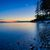# New to Qlik Sense

If you’re new to Qlik Sense, start with this Discussion Board and get up-to-speed quickly.

Announcements
Our May 2021 end-to-end product release from Data Integration to Data Analytics is out! READ DETAILS
cancel
Showing results for
Did you mean:Creator

## Load script equivalent of occurrence count in chart expression

Hi,

I'm wondering whats the load script equivalent of  the below Chart Expression?

if(count(Total <PBI> Incident_ID)<=1,1,
if(count(Total <PBI> Incident_ID)> 1 and count(Total <PBI> Incident_ID) <=5,'2-5',
if(count(Total <PBI> Incident_ID)>=6 and count(Total <PBI> Incident_ID) <=10,'6-10',
if(count(Total <PBI> Incident_ID)>10,'10+'))))

As I cant seem to add the above Expression as dimension.

1 Solution

Accepted SolutionsCreator
Hi,

You can achieve this using Group by at backend.
Try something like this..

PBI,
if(count(Incident_ID)<=1,1,
if(count(Incident_ID)> 1 and count(Incident_ID) <=5,'2-5',
if(count(Incident_ID)>=6 and count(Incident_ID) <=10,'6-10',
if(count(Incident_ID)>10,'10+')))) as IncidentCount
Resident TableName Group by PBI;
2 RepliesCreator
Hi,

You can achieve this using Group by at backend.
Try something like this..

PBI,
if(count(Incident_ID)<=1,1,
if(count(Incident_ID)> 1 and count(Incident_ID) <=5,'2-5',
if(count(Incident_ID)>=6 and count(Incident_ID) <=10,'6-10',
if(count(Incident_ID)>10,'10+')))) as IncidentCount
Resident TableName Group by PBI;MVP & Luminary

You need an aggr() to create a calculated dimension which includes an aggregation-function, for example with something like this:

aggr(pick(match(count(Total <PBI> Incident_ID), 0, 1, 2, …, 11, …), '1', '1', '2-5', …, '10+', …), PBI)

The suggested pick(match()) is just a replacement for multiple if's to get a better readability of the expression and often also an improved performance.

- Marcus